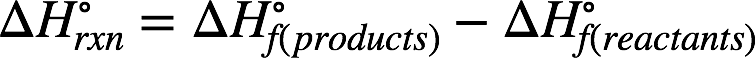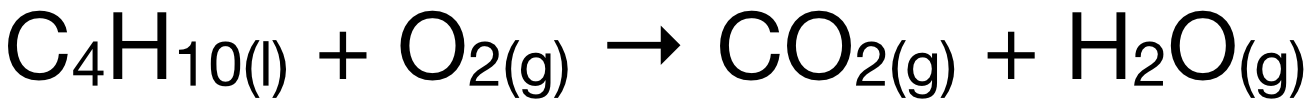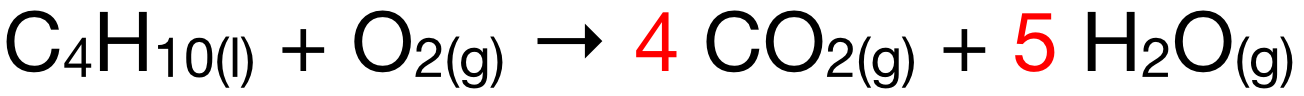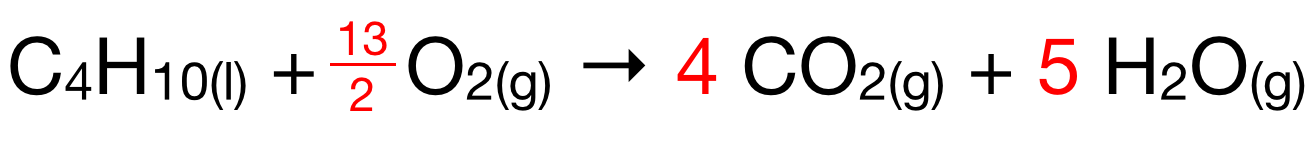# Problem: Calculate the enthalpy of combustion of butane, C 4H10(g) for the formation of H2O(g) and CO2(g). The enthalpy of formation of butane is −126 kJ/mol.

###### FREE Expert Solution

Recall: The enthalpy of reaction (ΔH˚rxn) can be calculated from the reactants' and products' enthalpy of formation (∆H˚f) using the equation:In the combustion, C4H10(l) reacts with O2(g) to form H2O(g) and CO2(g). The chemical equation is:This equation is not yet balanced. To balance it, add a coefficient of 4 to CO2 to balance the number of C. Add a coefficient of 5 to H2O to balance the number of H. The equation currently looks like this:The equation currently has 2 O in the reactant side and 13 O in the product side. We add a coefficient of 13/2 to O2 to balance the number of O. The balanced equation is:95% (172 ratings)###### Problem Details

Calculate the enthalpy of combustion of butane, C 4H10(g) for the formation of H2O(g) and CO2(g). The enthalpy of formation of butane is −126 kJ/mol.